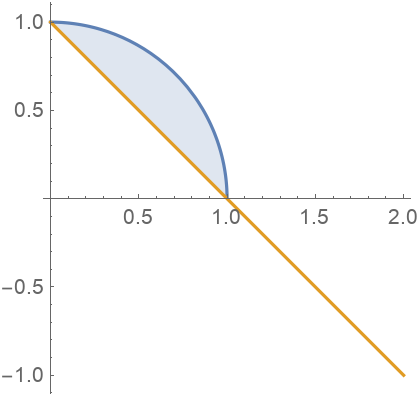Function Repository Resource:

AreaBetweenCurves

Find the area between two plane curves

Contributed by: Wolfram|Alpha Math Team
 ResourceFunction["AreaBetweenCurves"][{f,g},{x,xmin,xmax}] finds the area of the enclosed region between the functions f(x) and g(x) over the interval xmin < x < xmax.

Details and Options

ResourceFunction["AreaBetweenCurves"] works with real‐valued functions over the Cartesian coordinate system.
The area between f(x) and g(x) is defined as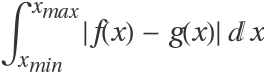.
When f(x) ≥ g(x), the area between the two curves is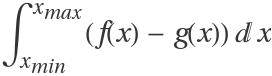.
When f(x) and g(x) only meet at x=xmin and x=xmax, the area is taken to be that of the enclosed region.
When f(x) and g(x) do not meet at x=xmin or x=xmax, the boundary of the enclosed region will contain vertical line segments joining the curves.
When f(x) and g(x) intersect for some xmin < x < xmax, the area will be that of multiple enclosed regions.
The following option can be given:
 assumptions on parameters

Examples

Basic Examples

Find the area between two curves:

 In:=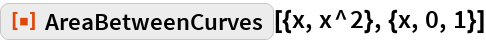Out=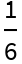In:=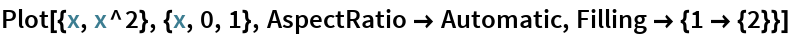Out=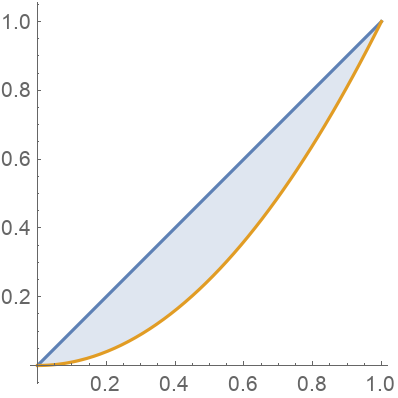Scope

Find the area of the region enclosed by two curves:

 In:=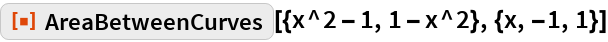Out=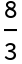In:=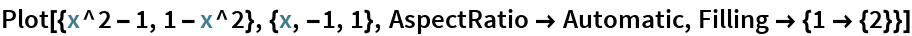Out=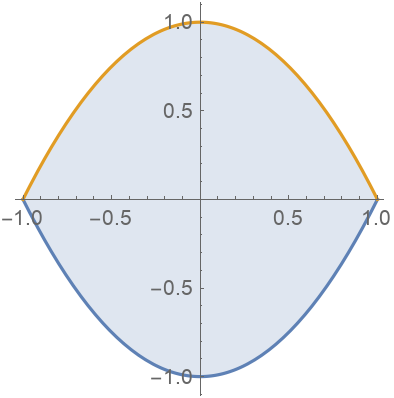Where the curves do not meet:

 In:=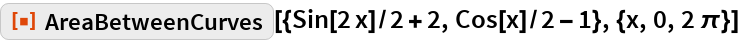Out=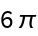In:=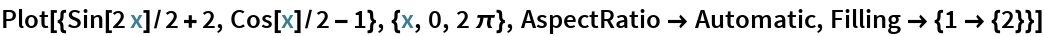Out=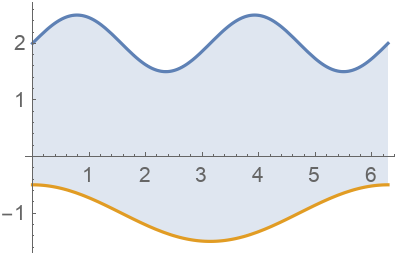With multiple enclosed regions:

 In:=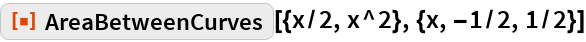Out=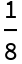In:=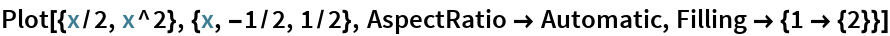Out=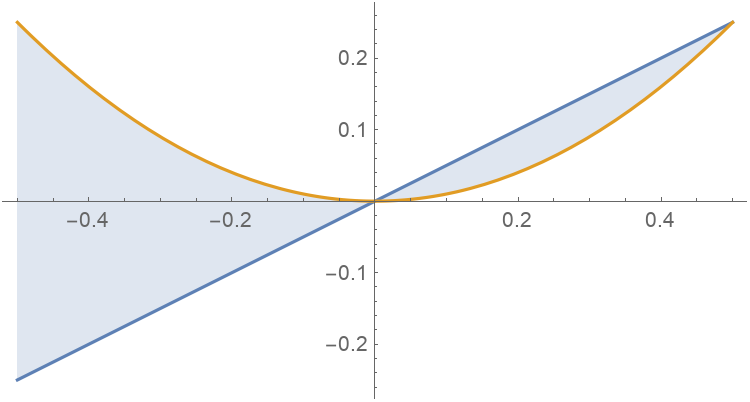Between curves containing parameters:

 In:=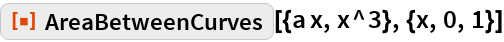Out=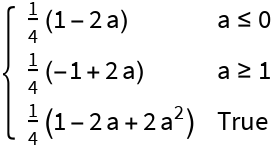Generalizations and Extensions

Find the area over an unbounded interval:

 In:=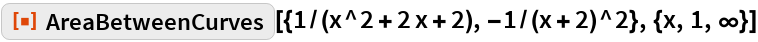Out=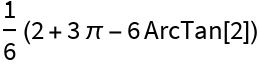In:=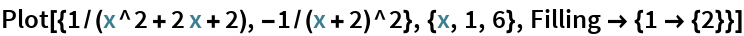Out=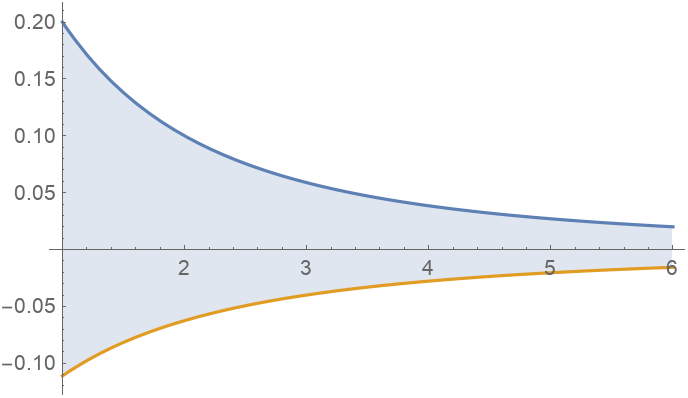Curves with discontinuities over intervals:

 In:=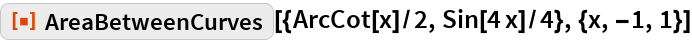Out=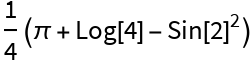In:=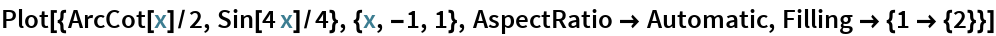Out=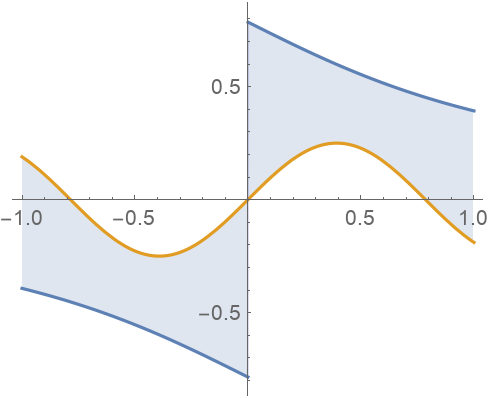With singularities:

 In:=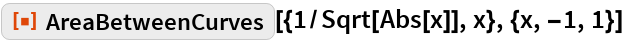Out=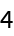In:=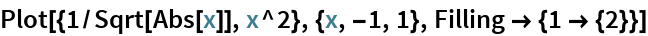Out=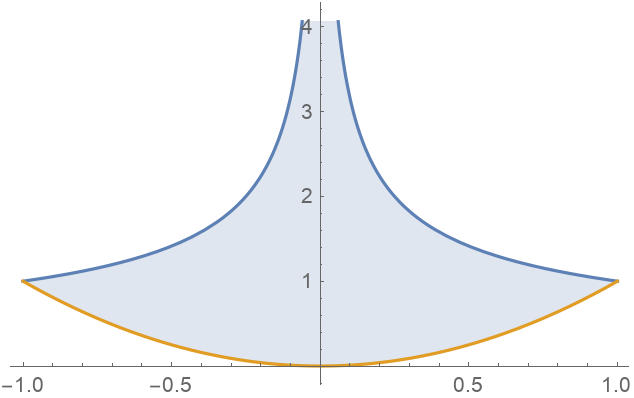Options

Assumptions

The result may be conditioned on parameters:

 In:=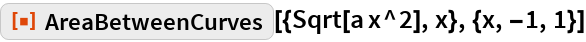Out=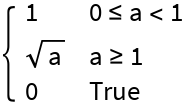Make an assumption about the parameter:

 In:=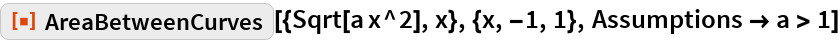Out=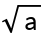Applications

Compute the area of a disk:

 In:=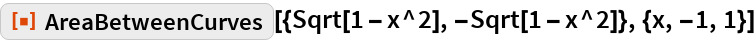Out=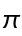In:=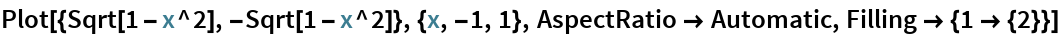Out=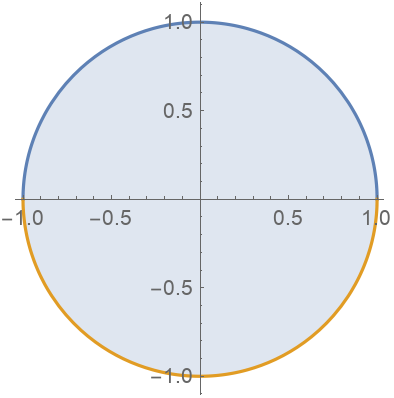Cavalieri's principle states that the area between two curves does not change when each curve is shifted by the same amount:

 In:=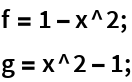In:=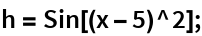In:=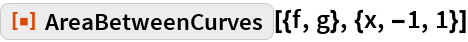Out=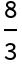In:=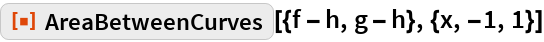Out=In:=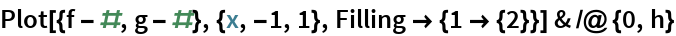Out=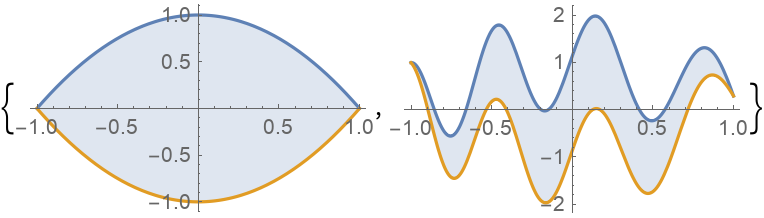The population growth of a region is currently growing at a rate of 35.208 ⅇ0.0083 t hundred people per year. It is thought that a large spike in employment opportunities can drop the growth rate to 24.098 ⅇ0.0071 t hundred people per year over the next 5 years. Find how many fewer people will be born if such a spike occurs:

 In:=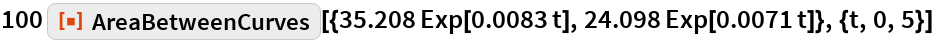Out=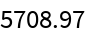Properties and Relations

Area is always non-negative:

 In:=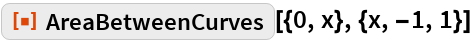Out=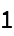The order in which the curves are specified does not matter:

 In:=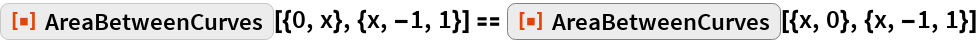Out=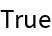Find the area of multiple enclosed regions:

 In:=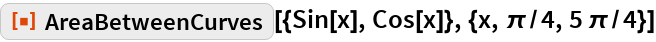Out=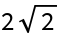Sum over each enclosed region instead:

 In:=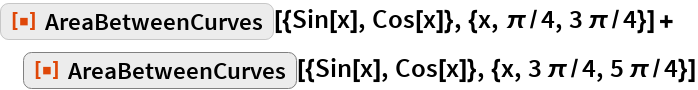Out=In:=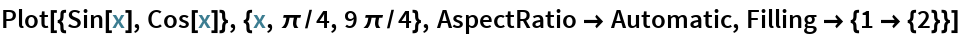Out=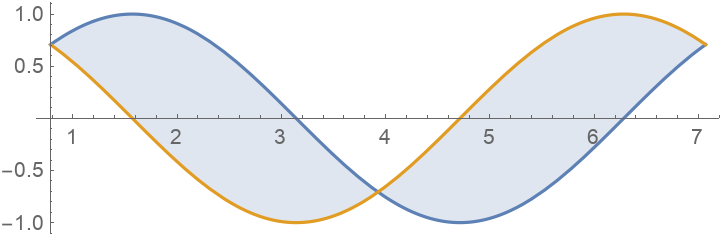The area between two curves is the integral of the absolute value of their difference:

 In:=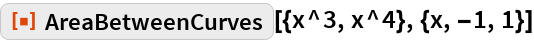Out=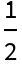In:=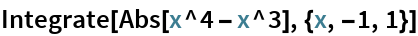Out=Possible Issues

The area between curves can be unbounded:

 In:=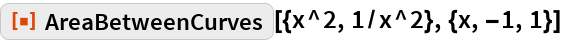Out=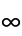Only real-valued components of curves are considered:

 In:=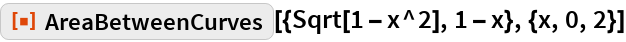Out=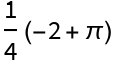In:=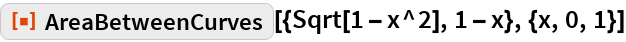Out=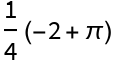In:=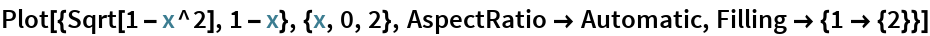Out=